# Weigh in total

I put 3/5 kg of grapes into a box which is 1/4kg in weight. How many kilograms do the grapes and the box weigh in total?

Correct result:

m =  0.85 kg

#### Solution: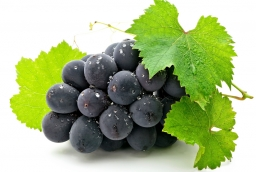We would be pleased if you find an error in the word problem, spelling mistakes, or inaccuracies and send it to us. Thank you!Tips to related online calculators
Need help calculate sum, simplify or multiply fractions? Try our fraction calculator.

## Next similar math problems:

• Water in body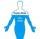Water in the human organism of all the compounds represented, constitutes about 3/5 of the total weight. How many kilograms of water is in your body when you weigh 51 kg?
• Birthday party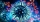For her youngest son's birthday party, mother bought 6 3/4 kg of hotdog and 5 1/3 dozens bread rolls. Hotdogs cost 160 per kilogram and a dozen bread roll costs 25. How much did she spend in all?
• Children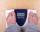Margaret and Zdena weigh the same and Petra 3 kg more. Together weigh 156 kg. How much do they weigh?
• Center of gravityThe mass points are distributed in space as follows - specify by coordinates and weight. Find the center of gravity of the mass points system: A1 [1; -20; 3] m1 = 46 kg A2 [-20; 2; 9] m2 = 81 kg A3 [93 3/4 + 2 3/5 + 5 1/2 Show your solution.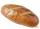5 of the same bread has the same weight as three bread and 4 kg of fruit. What weight has one bread?
• Dividing walnuts into crates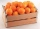There are 8 and 2 over 3 pounds of walnuts in a container, which will be divided equally into containers that hold 1 and 1 over 5 pounds. This would fill n and 4 over 18 containers. What is n?
• Farmers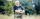Farmers loaded into a truck of fruit and vegetables intended for the store. 10 boxes of 5 kg pears, 8 boxes of 6 kg plums, 7 boxes 9 kg of carrots and 10 bags of 50 kg of potatoes. How many kilograms of fruit and vegetables loaded in total? How many kg co
• Brick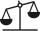Isosceles scale has on one side all brick and second weight 1 kg and 1/4 of brick. The balance is in equilibrium. What is the weight of a brick?
• ChestnutsThree divisions of nature protectors participated in the collection of chestnut trees.1. the division harvested 1250 kg, the 2nd division by a fifth more than the 1st division and the 3rd division by a sixth more than the second division. How many tons of
• Sales storesThe first sales store passed the 1/3 and the second 2/5 of the total amount of goods.In third store passed the 2/3 rest of the goods. The remaining 40 kg of goods put into fourth store. How many kilograms of goods pass to a third store?
• Evaluate expressionEvaluate expression using BODMAS rule: 1 1/4+1 1/5÷3/5-5/8
• Weight of airWhat is the weight of air in the living room measuring width 10 m length 3 m and height 3.3 m? Air density is ρ = 1.2 kg/m3.
• How many 3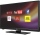How many hours the Andersons watched TV in all Wednesday 3/1 hr Thursdays 2/3 hr Friday 4/5 hr Saturday 3/4 hr
• Barrel 4Barrel of water weighs 63 kg. After off 75% water, the weight of the barrel with water is 21 kg. How many kg weigh empty barrel and how many kgs water in it?
• Cast brass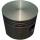Brass cast weight was 3.84 kg, it had turned into piston weight 3.491 kg. How many grams of brass was turned off?
• Crates 2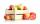In 6 crates is 45 kg of apples In  5 crates is equally In 1 in crate is 3 kg more How many kilograms in each crate?